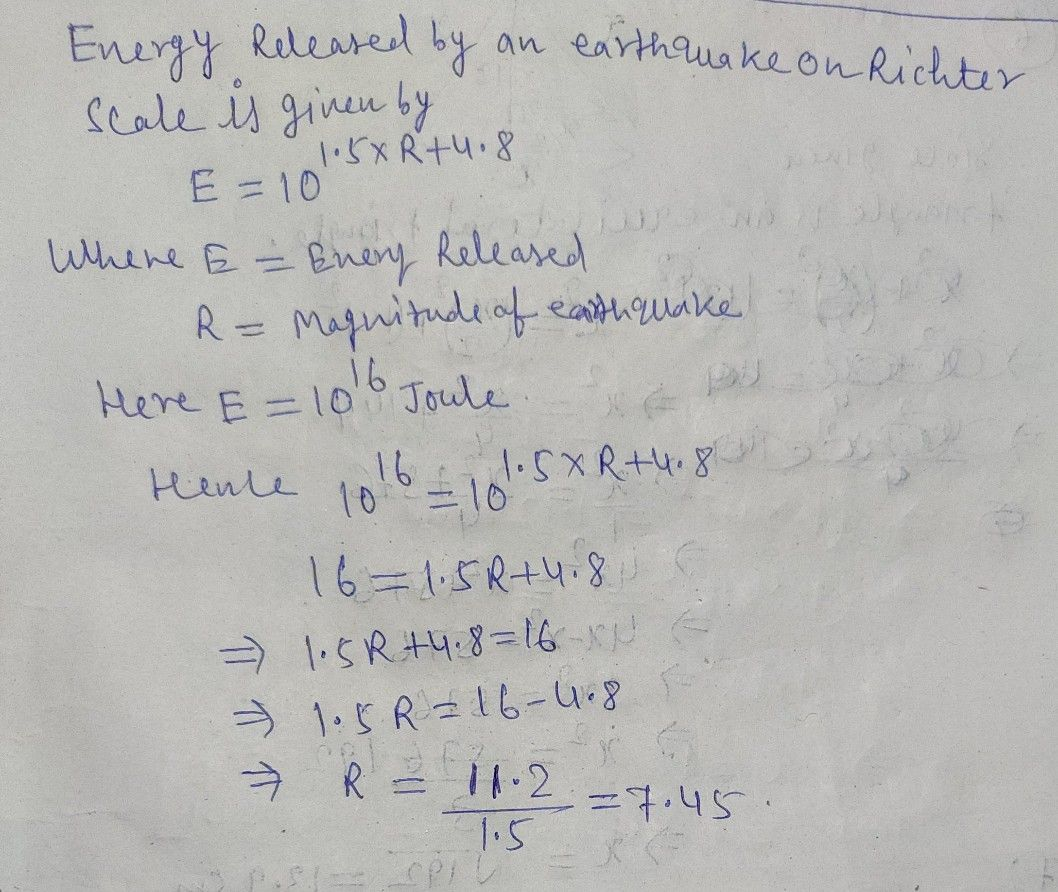Symbol
Problem$:1.$ The energy released by $an$ earthquake $1s$ $10^{1}$ joules. o $n\right)$ $a\right)$ Compute for the magnitude of the earthquake in a Richter scale. (show solution) $b\right)$ How many times more energy does this earthquake release than that of the reference earthquake? (show solution) $n\right)$ niwolio ed lo dass ovloci yivi
10th-13th grade
Other
SolutionQanda teacher - RanjeetStudent
thankyou sirrr